In :
# %load /Users/facai/Study/book_notes/preconfig.py
%matplotlib inline

import matplotlib.pyplot as plt
import seaborn as sns
sns.set(color_codes=True)
#sns.set(font='SimHei')
plt.rcParams['axes.grid'] = False

import numpy as np

#from IPython.display import SVG
def show_image(filename, figsize=None, res_dir=True):
if figsize:
plt.figure(figsize=figsize)

if res_dir:
filename = './res/{}'.format(filename)



# Chapter 9 Convolutional Networks¶

Convolution is a specialized kind of linear operation.

### 9.1 The Convolution Operation¶

\begin{align} s(t) &= \int x(a) w(t-a) \mathrm{d}a \\ &= (x \ast w)(t) \end{align}

where $x$ is the input, $w$ is the kernel, and $s(t)$ is referred to as the feature map.

Since convolution is commutative,

\begin{align} S(i, j) &= (I \ast K)(i, j) &= \sum_m \sum_n I(m, n) K(i - m, j - n) \\ &= (K \ast I)(i, j) &= \sum_m \sum_n I(i - m, j - n) K(m, n) \end{align}

Many machine learning libraries implement cross-correlation but call it convolution.

Discrete convolution can be viewed as multiplication by a matrix.

In :
show_image("fig9_1.png", figsize=(8, 8))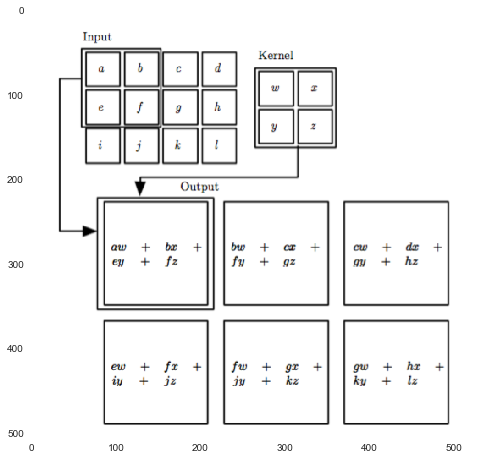### 9.2 Motivation¶

Convolution leverages three important ideas:

• sparse interactions: fewer parameters
• parameter sharing: tied weights
• equivariant representations:
a function $f(x)$ is equivariant to a funtion $g$ if $f(g(x)) = g(f(x))$.
In :
show_image("fig9_5.png", figsize=(8, 5))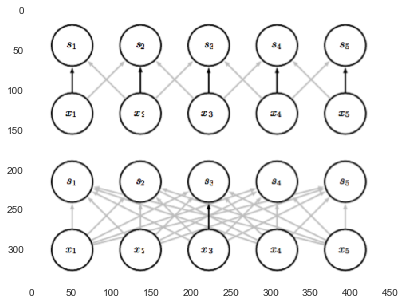### 9.3 Pooling¶

A pooling function replaces the output of the new at a certain location with a summary statistic of the nearby outputs.

popular pooling functions:

• max
• average
• L2 norm
• weighted average

strong prior: function must be invariant to small translations.

In :
show_image("fig9_7.png", figsize=(10, 8))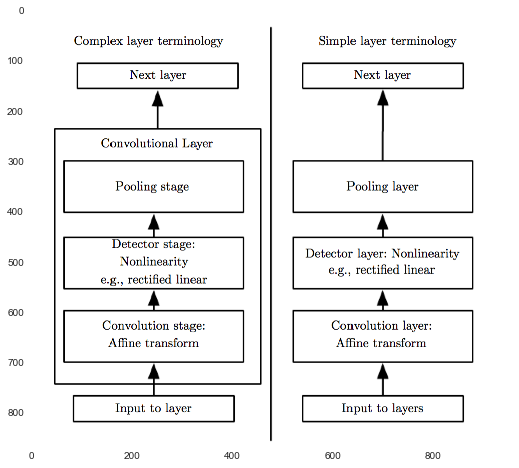In :
show_image("fig9_9.png", figsize=(10, 8))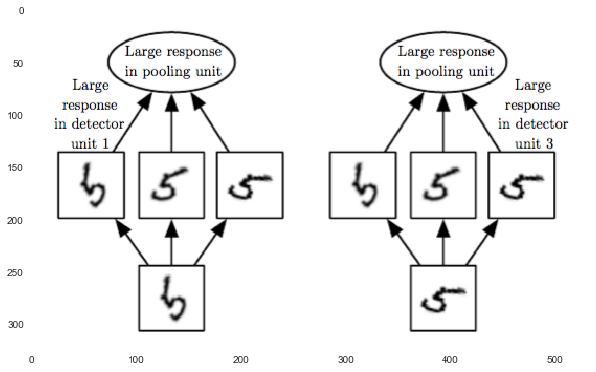### 9.4 Convolution and Pooling as an Infinitely Strong Prior¶

Prior: weak or strong <== how concentrated the probability density in the prior is.

We can image a convolutional net as being similar to a fully connected net, but with an infinitely strong prior over its weights: the weights for one hidden unit must be identical to the weights of its neighbor, but shifted in space.

convolution and pooling can cause underfitting.

### 9.5 Variants of the Basic Convolution Function¶

4-D tensors: (batch_size, height, width, channels)

• valid convolution: m - k +1 = m - (k - 1)
• same convolution: m，补零至核中心
• full convolution: m + k - 1，补零至核边角

see details below.

In :
show_image("matlab_conv_2d.png", figsize=(12, 8))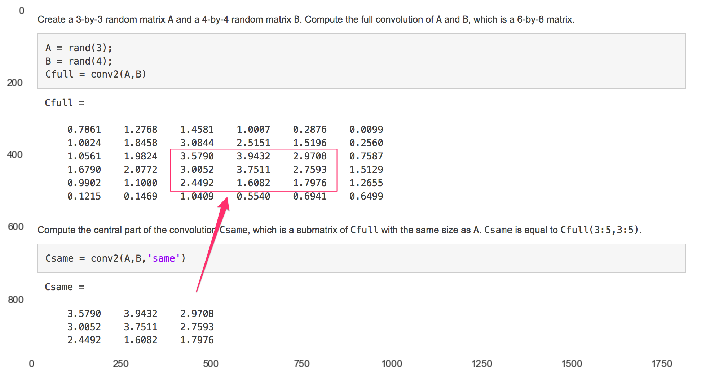In :
A = np.random.rand(3, 3)
A

Out:
array([[ 0.35212801,  0.37922789,  0.65163256],
[ 0.59534513,  0.74098237,  0.42971692],
[ 0.73934346,  0.73590775,  0.10633755]])
In :
B = np.random.rand(4, 4)
B

Out:
array([[ 0.20413973,  0.24286469,  0.83290138,  0.30052197],
[ 0.93643405,  0.41111035,  0.60742698,  0.0043744 ],
[ 0.20255814,  0.3365866 ,  0.26242548,  0.85468478],
[ 0.64287861,  0.38078144,  0.22940042,  0.62623427]])
In :
def gen_kernel_fn(kernel):
def kernel_fn(a, x_start, y_start):
x_size, y_size = kernel.shape
a_slice = a[x_start:x_start+x_size, y_start:y_start+y_size]
return (a_slice * kernel).sum()
return kernel_fn

def calc_conv2d_res_size(a, kernel):
res_x_size = a.shape - kernel.shape + 1
res_y_size = a.shape - kernel.shape + 1
return res_x_size, res_y_size

def conv2d(a, kernel):
kernel_fn = gen_kernel_fn(kernel)

res_x_size, res_y_size = calc_conv2d_res_size(a, kernel)
res = np.zeros((res_x_size, res_y_size))
for x in range(res_x_size):
for y in range(res_y_size):
res[x, y] = kernel_fn(a, x, y)
return res

In :
# valid convolution
conv2d(B, A)

Out:
array([[ 2.25524107,  1.82679291],
[ 2.14415575,  1.6570156 ]])
In :
def calc_2d_pad_width(target_size, real_size):
pad_x_width = (target_size - real_size) / 2
pad_y_width = (target_size - real_size) / 2

In :
def zero_pad_and_conv2d(a, kernel, target_size):
res_size = calc_conv2d_res_size(a, kernel)


In :
# same convolution
same_conv_size = B.shape

Out:
array([[ 0.98847255,  1.71888185,  1.64232006,  1.17086158],
[ 1.29107212,  2.25524107,  1.82679291,  1.59511597],
[ 1.43133448,  2.14415575,  1.6570156 ,  1.63554738],
[ 0.93613643,  1.13343863,  1.44076255,  1.01712864]])
In :
# full convolution
full_conv_size = [x1 + x2 for (x1, x2) in zip(B.shape, A.shape)]
print("full convolution size: {}".format(full_conv_size))

full convolution size: [7, 7]

Out:
array([[ 0.02170772,  0.17605365,  0.41822408,  0.82445577,  0.83695663,
0.22218895],
[ 0.1873004 ,  0.98847255,  1.71888185,  1.64232006,  1.17086158,
0.18214847],
[ 0.55696519,  1.29107212,  2.25524107,  1.82679291,  1.59511597,
0.74033208],
[ 0.76561571,  1.43133448,  2.14415575,  1.6570156 ,  1.63554738,
0.97337498],
[ 0.40824929,  0.93613643,  1.13343863,  1.44076255,  1.01712864,
0.67378397],
[ 0.41892063,  0.49192708,  0.52026328,  0.62915348,  0.31826381,
0.22051462]])
##### Comparison of local connections, convolution, and full connections.¶

convolutional layers $\to$ tiled convolution $\to$ locally connected layer

In :
show_image("fig9_16.png", figsize=(10, 8))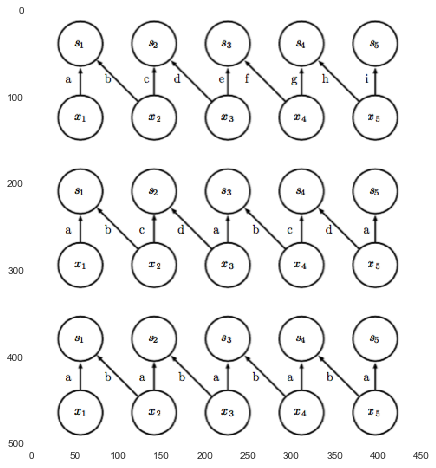compute gradients: See Goodfellow (2010) for a full derivation of the equations in the fully general multi-dimensional, multi-example case.

### 9.6 Structured Outputs¶

Convolutional networks => output a high-dimensional, structured object. For example, to label every pixel in an image.

### 9.7 Data Types¶

Convolutional networks can porcess inputs with varying spatial extents which is the same kind of observations.

### 9.8 Efficient Convolution Algorithms¶

• Fourier transform: point-wise multiplication in frequency domain.
• Sometimes d-dimensional kernel is separable.

### 9.9 Random or Unsupervised Features¶

Today, most convolutinoal networks are trained in a purely supervised fashion.

### 9.11 Convolutional Networks and the History of Deep Learning¶

In [ ]: